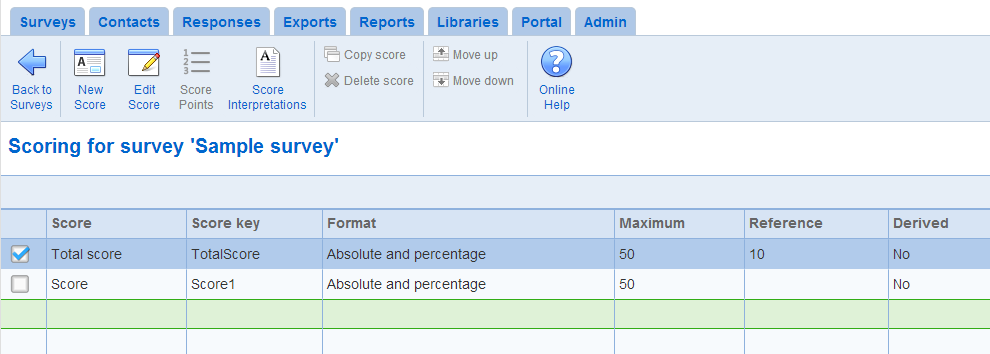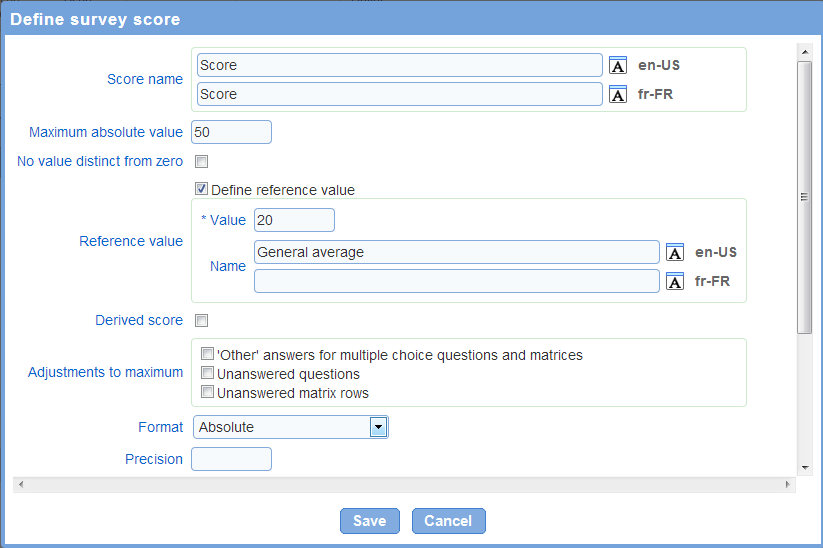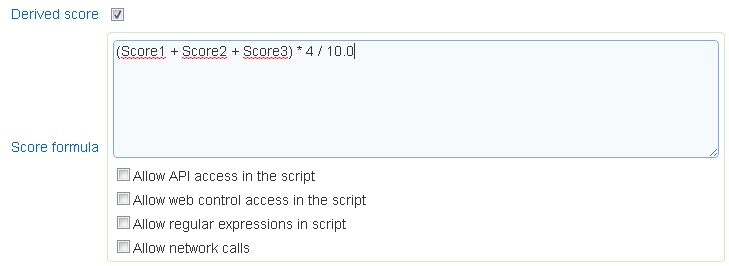The survey scores tab allow the definition of the scores for the survey. A survey has 1 total score and as many partial scores as desired. By definition, the total score is the sum of the partial scores.

Each partial score is a assigned a system generated key of the form ScoreN (where N is a whole number). The key can be used to embed the score in text such as the completion page, the completion email, or in single response reports.

Note that since the total score is computed from the partial scores, points for each question cannot be defined for the total score.• New Score: To create a new score for the survey, use tool New.

See Add/Setup score below for a discussion of the fields for a score.

• Edit score: To modify an existing score, use the Setup tool.

See Add/Setup score below for a discussion of the fields for a score.

• Score points: Allows to define the points for the score based on the questions in the survey

• Copy score: Creates a copy of the selected score. The points assigned for the score from each question in the survey are copied also.

• Delete score: Deletes the selected score. Once deleted, a score cannot be recovered.

• Move (up, down): To change the position of a score, use the Move up or Move down tools. The order of the score determines how they are displayed in report elements charts and statistics that include the score.

Note that field "Score key" in the table defines the tag to use in the completion email or the completion page to include the score.The following fields are available for a score:

• Score name: defines the name of the score as it will be shown to respondents and in reports. The score name is required for each of the languages for the survey.

Note that using the calculate button computes an estimated value for the maximum as a one-time operation. Changes to the point structure for the survey after the estimation is performed will not be reflected in the maximum score.

• No value distinct from zero: Determines if the absence of value is handled distinctly from a score value of zero.  The option specifies whether the score should be shown either as '0' or as blank when it does not have a value (e.g., when none of the questions contributing to the score are answered by the respondent). The option applies to exports, and pipes in the completion email, the completion message, and reports. If the option is checked and the score does not have an assigned value then the score will be shown as blank.  If the option is not checked and the score does not have an assigned value then the score will be shown as 0.

When a chart includes more than one reference value, there will be one data series for each unique name for the reference values included. For example, in a bar chart with 3 scores, each with a reference value with name 'General population', there will be one series in the chart for the score and one series for the three reference values. The chart legend will contain two entries, one for the scores and one for the reference values. If the name of one of the three reference values is changed to 'Overall' then there will be one legend entry for the two reference values with name 'General population' and one legend entry for the series for reference 'Overall'. For more details on series in charts, see Reports - charting.

• Adjustments to maximum: the value for the maximum score can be adjusted to a lower value automatically based on the respondent's answers. Supported adjustments include:

1. 'Other' answers for multiple choice questions and matrices: the maximum score will be decreased by the maximum point value for multiple choice question and matrices where the respondent chooses the 'Other/NA' answer.

2. Unanswered questions: the maximum score will be decrease by the maximum point value for questions for which the respondent does not provide an answer.

3. Unanswered matrix rows: the maximum score will be decrease by the maximum point value for matrix row for which the respondent does not provide an answer.

Note that due to adjustments, it is possible for the maximum score to reach 0 or a negative value.

• Format: defines how the score will be displayed to respondents and in reports.

1. Absolute: the score is displayed as a point value.

2. Percentage: the score is displayed as a percentage. The percentage is the point value attained by the respondent divided by the maximum value for the score after adjustment (see above for a discussion of adjustments). If the maximum point value is 0, then the percentage will not be calculated and the score will not be shown.

3. Absolute and percentage: both the absolute point value and the percentage (see above) will be shown.

Note that if the point value for the respondent's answer is not defined (i.e., none of the answers have a point value defined for them), then the score will not be displayed. Additionally, the total score can only be defined in absolute format. The total score cannot be expressed as a percentage.

• Precision: Specifies the number of digits after the decimal point to display when the score is included in a report or in the completion page for the survey. The precision option is particularly useful for scores expressed as percentages  and for derived scores. For example, if the value of a score is 34.4163 and the precision is set to 1, the score value will be displayed as 34.4. Note that independently of this option, the precision for scores displayed in charts for report can be affected by the 'Number precision' option for the chart (See: Reports - Charting).

Derived score

A score can be defined as a formula from other scores. If the formula, the keys assigned to the scores are used to reference the scores. Note that if the formula is invalid (e.g., parentheses are not balanced or there is a division by 0), then the page that uses the score will produce an error.

Creation of derived scores requires the "Scripting" role.